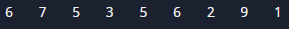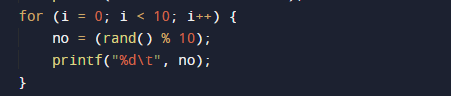# C Program to Generate Random Numbers

In this tutorial you will learn about the C Program to Generate Random Numbers and its application with practical example.

In this tutorial, we will learn to create a C program that  will generate  a random number  using C programming.

## Prerequisites

Before starting with this tutorial we assume that you are best aware of the following C programming topics:

• Basic C programming.
• Operators.
• Looping statements
• For Loop.
• Basic input/output.
• Inbuilt library function.

## What Is Random number?

Random number is are set of digits (i.e., 0, 1, 2, 3, 4, 5, 6, 7, 8, 9) arranged in irregular order.As you can see here some random number between 1 to 10 are shown in above image we will use here a rand() function to generate 10 different random number in our our program let us see how to do that.

## C Program to Generate Random Numbers.

Here in our program we are going to use rand() inbuilt function which is define in header file stdlib.h with the help of this function we genrate 10 differnet random number let have a look at program.

## OutputIn the above program, we have first declared and initialized a set variables required in the program.

• no = For holding random values
• i = for iteration.

First of all we declare two variable i for iteration and no  for holding value provided by rand() function. Here in this program we use rand() function to generate 10 random number in our program.In our program we use for loop to print Ten different random value using rand() function at multiple times.As we can see in above image that in each iteration the rand() function called every time gives us a new random number between 1-10 because we use range from 1-10 to print 10 different values if we want to print ten different values form 1-100  then with rand() function we have to use rand()%100 it will gives us ten different value between 1-100.

In this tutorial we have learn about the C Program to Generate Random Numbers and its application with practical example. I hope you will like this tutorial.